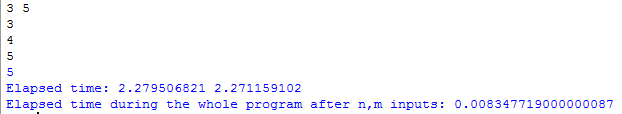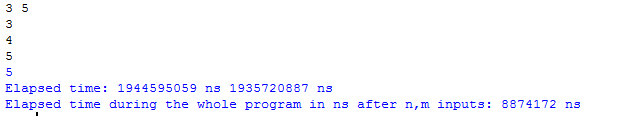Open in App
Not now

# time.perf_counter() function in Python

• Difficulty Level : Easy
• Last Updated : 23 Mar, 2022

The time module provides various time-related functions. We must import the time module before using perf_counter() so we can access the function without throwing any errors.
The perf_counter() function always returns the float value of time in seconds. Return the value (in fractional seconds) of a performance counter, i.e. a clock with the highest available resolution to measure a short duration. It does include time elapsed during sleep and is system-wide. The reference point of the returned value is undefined, so that only the difference between the results of consecutive calls is valid. In between this we can use time.sleep() and likewise functions.
Code #1: Understand the usage of the perf_counter

## Python3

 `# Python program to show time by perf_counter()``from` `time ``import` `perf_counter` `# integer input from user, 2 input in single line``n, m ``=` `map``(``int``, ``input``().split())` `# Start the stopwatch / counter``t1_start ``=` `perf_counter()` `for` `i ``in` `range``(n):``    ``t ``=` `int``(``input``()) ``# user gave input n times``    ``if` `t ``%` `m ``=``=` `0``:``        ``print``(t)` `# Stop the stopwatch / counter``t1_stop ``=` `perf_counter()` `print``(``"Elapsed time:"``, t1_stop, t1_start)`  `print``(``"Elapsed time during the whole program in seconds:"``,``                                        ``t1_stop``-``t1_start)`

Output:pref_counter_ns():
It always gives the integer value of time in nanoseconds. Similar to perf_counter(), but return time as nanoseconds.
Code #2: Usage of the perf_counter_ns and how to implement it.

## Python3

 `# Python program to show time by``# perf_counter_ns()``from` `time ``import` `perf_counter_ns` `# integer input from user, 2 input in single line``n, m ``=` `map``(``int``, ``input``().split())` `# Start the stopwatch / counter``t1_start ``=` `perf_counter_ns()` `for` `i ``in` `range``(n):``    ``t ``=` `int``(``input``()) ``# user gave input n times``    ``if` `t ``%` `m ``=``=` `0``:``        ``print``(t)` `# Stop the stopwatch / counter``t1_stop ``=` `perf_counter_ns()` `print``(``"Elapsed time:"``, t1_stop, ``'ns'``, t1_start, ``'ns'``)` `print``(``"Elapsed time during the whole program in ns after n, m inputs:"``,``       ``t1_stop``-``t1_start, ``'ns'``)`

Output:Compare both the outputs of the program as perf_counter() returns in seconds and pers_counter_ns() returns in nanoseconds.